﻿ 风载荷作用下的操纵性研究
 舰船科学技术2019, Vol. 41Issue (8): 48-54PDF

Research on maneuverability under wind load
WEI Ke-ke, GAO Xiao-peng
Department of Naval Architecture, Naval University of Engineering, Wuhan 430033, China
Abstract: In order to study the maneuverability of a surface ship under the certain wind load, the three-degree-of-freedom MMG maneuverability equation under still water and wind load is constructed based on the Matlab software platform. It is found that the simulation value is in good agreement with the test value by comparing the results of the numerical forecast in the still water with the test value,which proves the feasibility of the method.The numerical calculation of the maneuverability under wind load is carried out and compared with the numerical results of still water. The results show that: The rotational angular parameters such as the dimensionless rotation diameter, the dimensionless tactical diameter, the velocity drop, the dimensionless transverse distance are smaller than the theoretical forecast value in the hydrostatic, and the rotational angular velocity is basically the same as the theoretical value of the hydrostatic theory, and the dimensionless longitudinal distance are greater than the theoretical forecast value in the hydrostatic. And the Z-shaped motion parameters such as the initial prediction period, the transcendental time, the first transcendental angle and the second transcendental angle are larger than those of the hydrostatic theoretical forecast value. It can be seen that the wind load has a maneuverability influence to the surface ship when compared with still water, and the numerical forecasting method can provide reference for engineering application.
Key words: MMG     wind load     rotational motion     Z-shaped motion
0 引　言

1 操纵性运动模型 1.1 常规的MMG操纵性运动方程

MMG操纵性方程是一个通用的方程。该方程分别单独考虑船、桨、舵的水动力及其之间的相互干扰，方程的具体形式如下式：

 $\left\{ \begin{gathered} (m + {m_x})(\dot u + qw - vr) = X = {X_H} + {X_P} + {X_R}{\text{，}} \\ (m + {m_y})(\dot v + ur - pw) = Y = {Y_H} + {Y_R} + {Y_P} {\text{，}}\\ (m + {m_z})(w + pv - qu) = Z = {Z_H} + {Z_R} + {Z_P}{\text{，}}\\ {I_x}\dot p + ({I_z} - {I_y})qr = L = {L_H} + {L_R} + {L_P}{\text{，}} \\ {I_y}\dot q + ({I_x} - {I_z})pr = M = {M_H} + {M_R} + {M_P}{\text{，}}\\ {I_z}\dot r + ({I_y} - {I_x})pq = N = {N_H} + {N_R} + {N_P} {\text{。}}\\ \end{gathered} \right.$ (1)

 $\left\{ \begin{gathered} (m + {m_x})(\dot u - ur) = X = {X_H} + {X_P} + {X_R} {\text{，}}\\ (m + {m_y})(\dot v + ur) = Y = {Y_H} + {Y_R} + {Y_P}{\text{，}} \\ {I_Z}\dot r = N = {N_H} + {N_R} + {N_P}{\text{。}} \\ \end{gathered} \right.$ (2)
1.2 风载荷作用下的操纵性方程

 $\left\{ \begin{gathered} \left( {m + {m_x}} \right)(\mathop u\limits^ \bullet - ur) = X = {X_H} + {X_P} + {X_R} + {X_{{\rm{Wind}}}} {\text{，}}\\ \left( {m + {m_y}} \right)(\mathop v\limits^ \bullet + ur) = Y = {Y_H} + {Y_R} + {Y_{{\rm{Wind}}}}{\text{，}} \\ {I_z}\mathop r\limits^ \bullet = N = {N_H} + {N_R} + {N_P} + {N_{{\rm{Wind}}}}{\text{。}} \\ \end{gathered} \right.$ (3)
2 静水中操纵性预报与验证

2.1 静水中的回转运动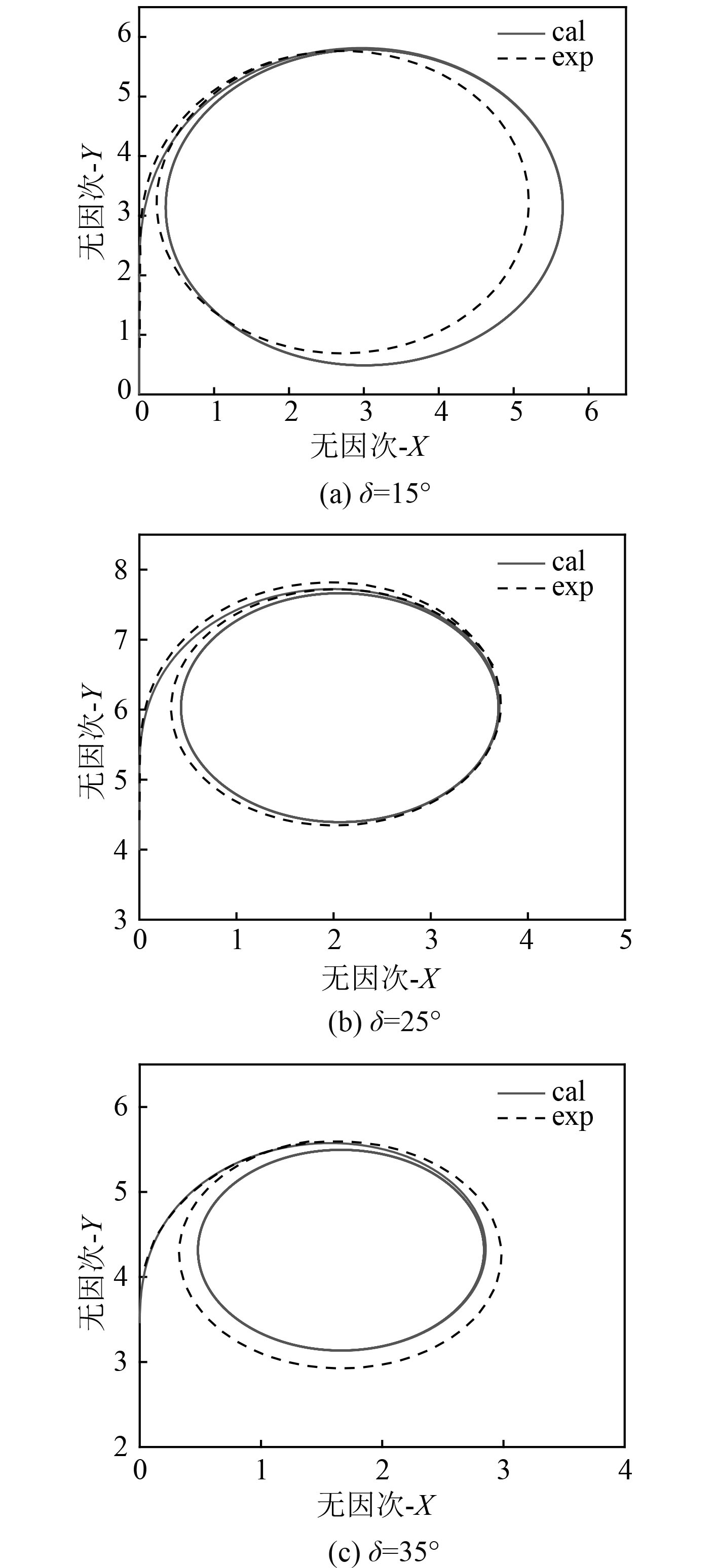图 1 不同舵角无因次回转轨迹与试验的对比 Fig. 1 Comparision of dimensionless rotating track to experimentation with different rudder表 1 回转运动中特征参数的结果对比 Tab.1 Comparison of the turning motion characteristic parameters
2.2 静水中的Z形运动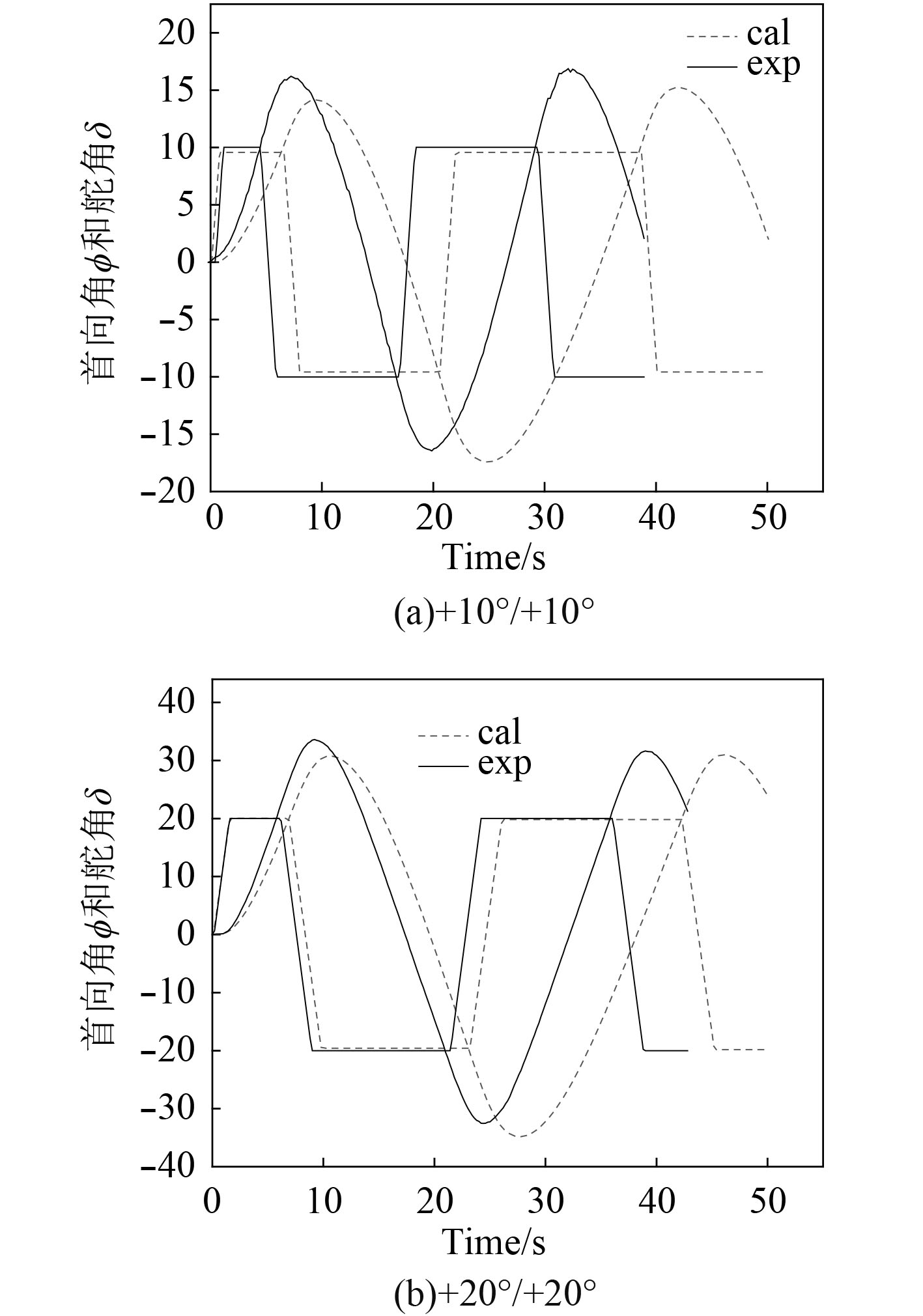图 2 不同舵角与首向角时历曲线的对比 Fig. 2 Comparision of the time curve with different rudder angle and course angle表 2 Z形运动中特征参数的结果对比 Tab.2 Comparison of the Zigzag motion characteristic parameters

3 风载荷作用下的操纵性预报

3.1 风载荷作用下的回转运动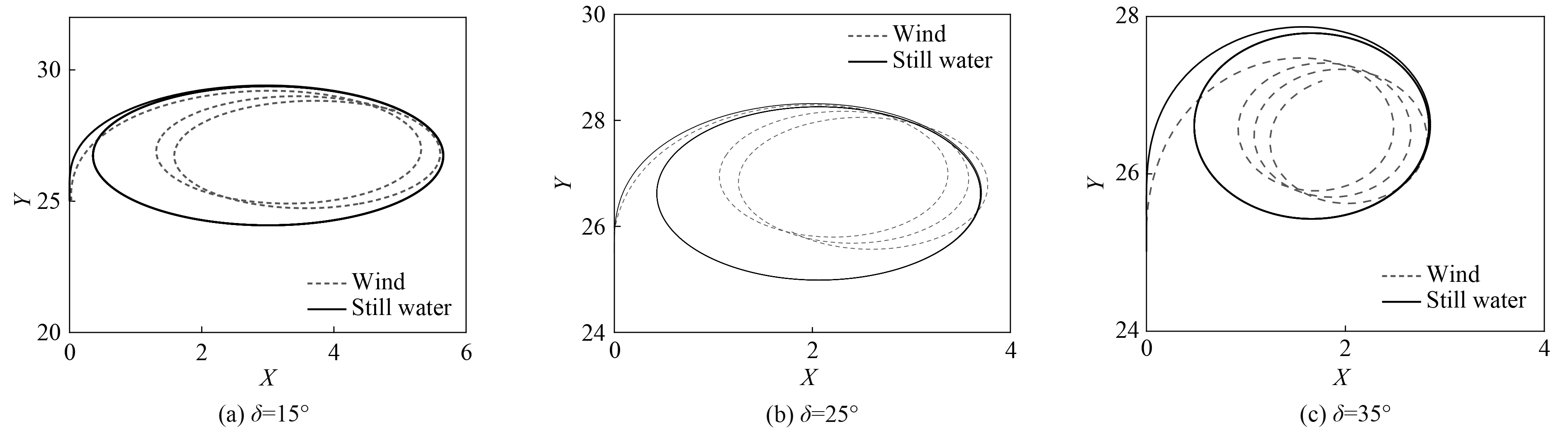图 3 不同舵角下无因次定常回转轨迹的对比 Fig. 3 Comparision of dimensionless rotating track with different rudder angle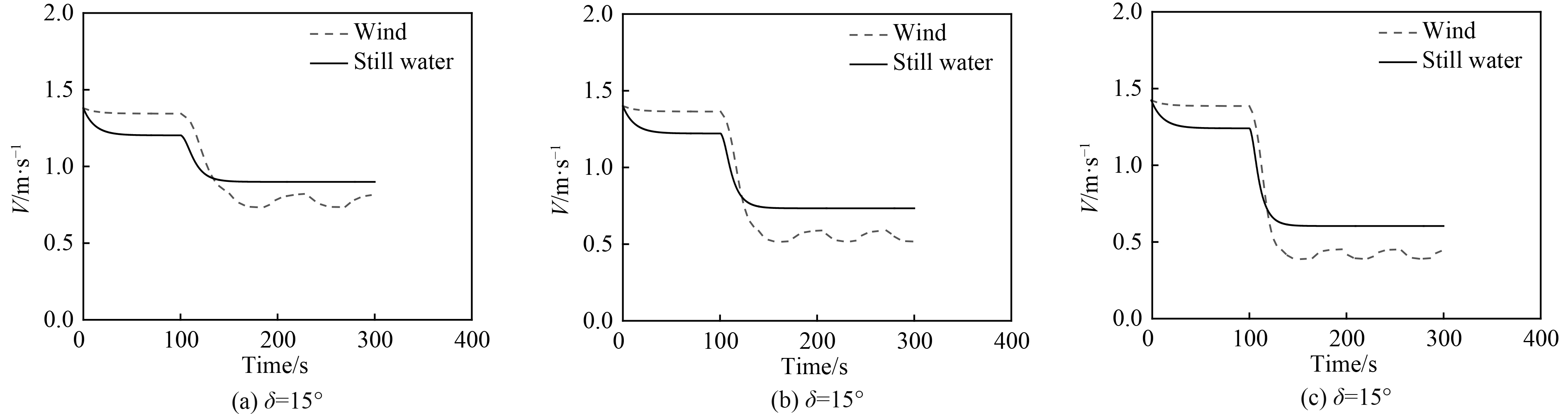图 4 不同舵角下航速 ${{V}}$ 的对比 Fig. 4 Comparision of velocity with different rudder angle

3.2 风载荷作用下的Z形运动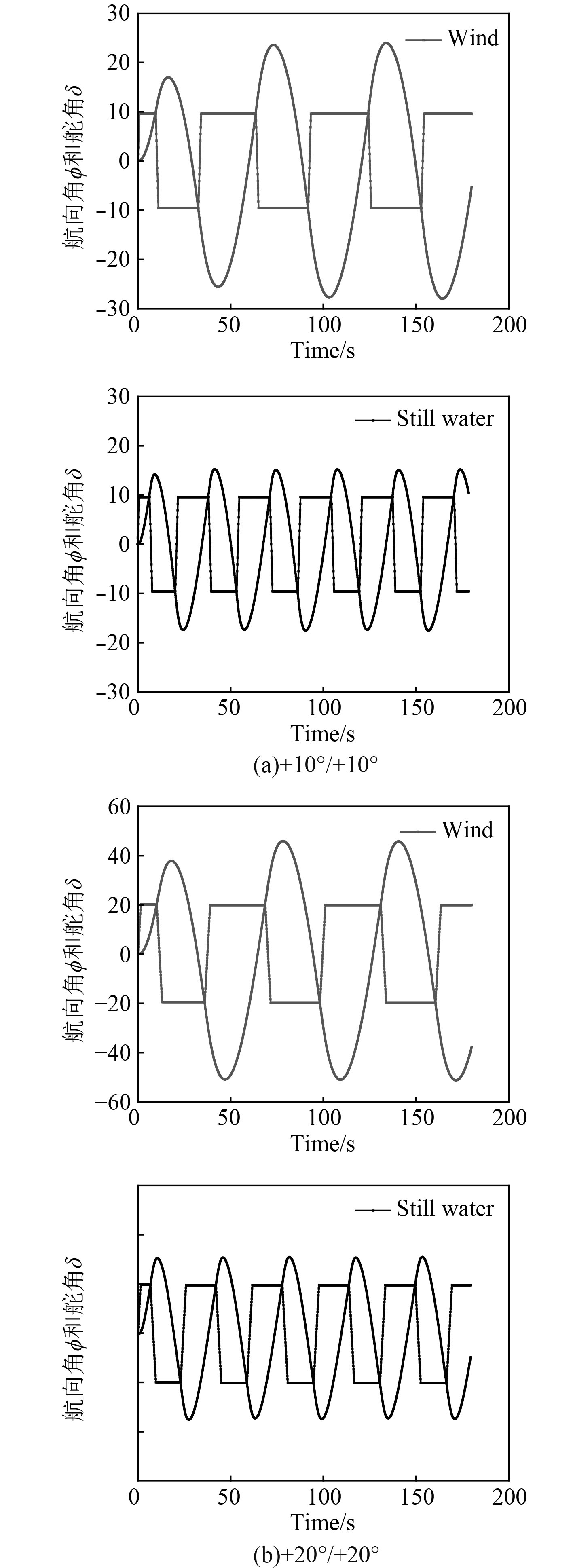图 5 不同舵角与首向角时历曲线的对比 Fig. 5 Comparision of the time curve with different rudder angle and course angle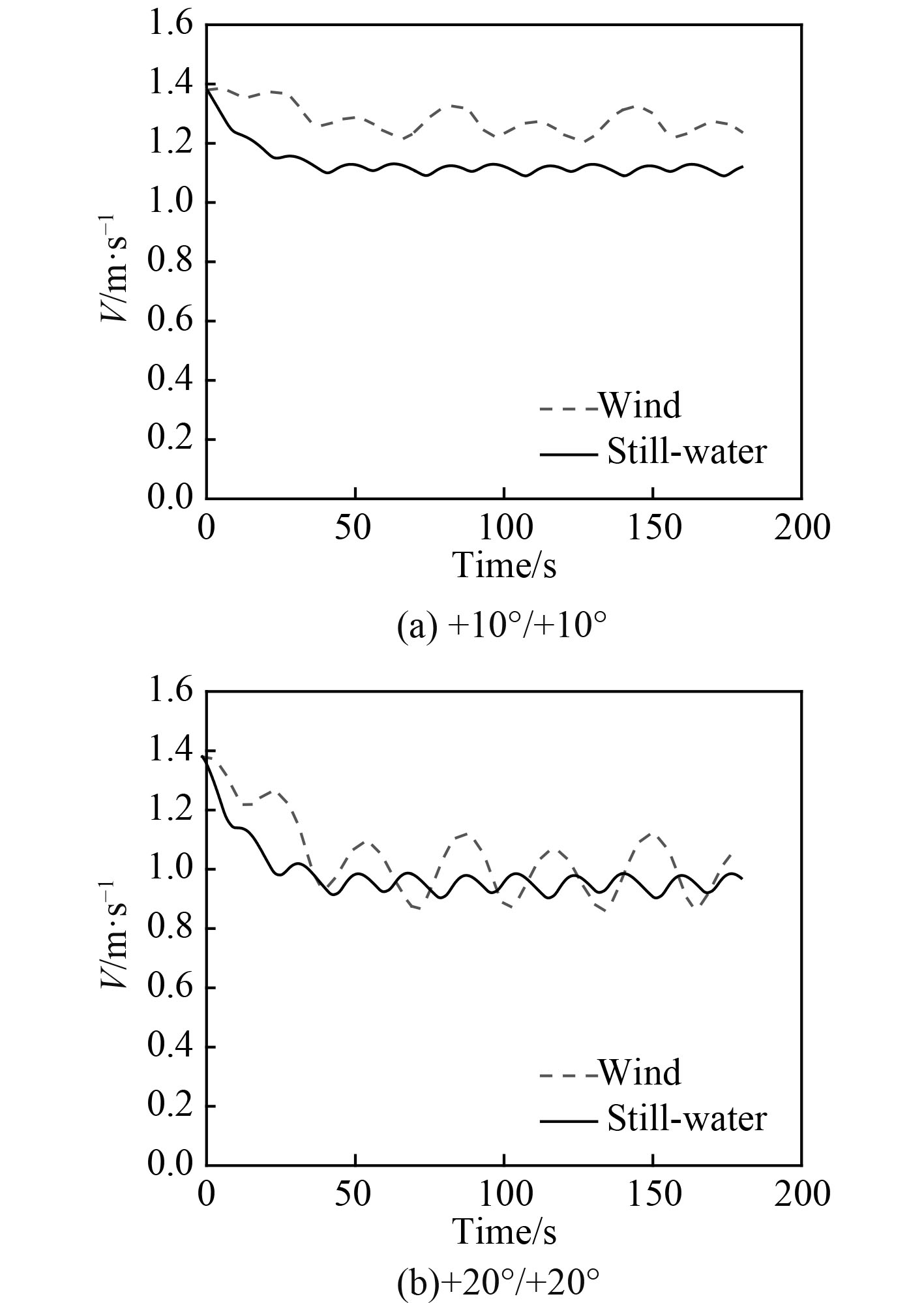图 6 不同舵角和首向角航速 ${{V}}$ 的对比 Fig. 6 Comparision of velocity with different rudder angle and course angle

3.3 对比分析 3.3.1 回转运动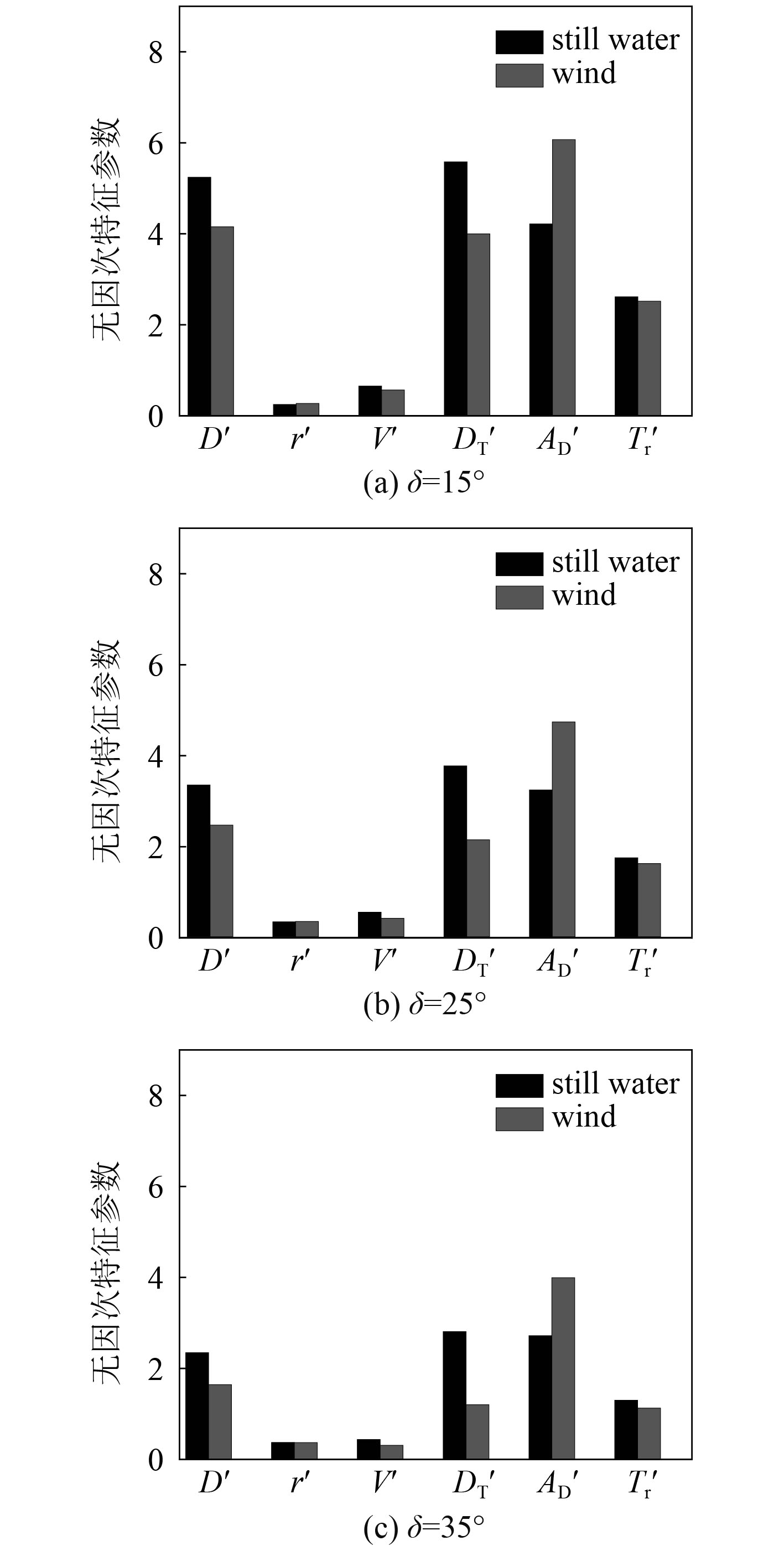图 7 不同舵角无因次回转特征参数的对比 Fig. 7 Comparison of dimensionless rotary characteristic parameters with different rudder angle

3.3.2 Z形运动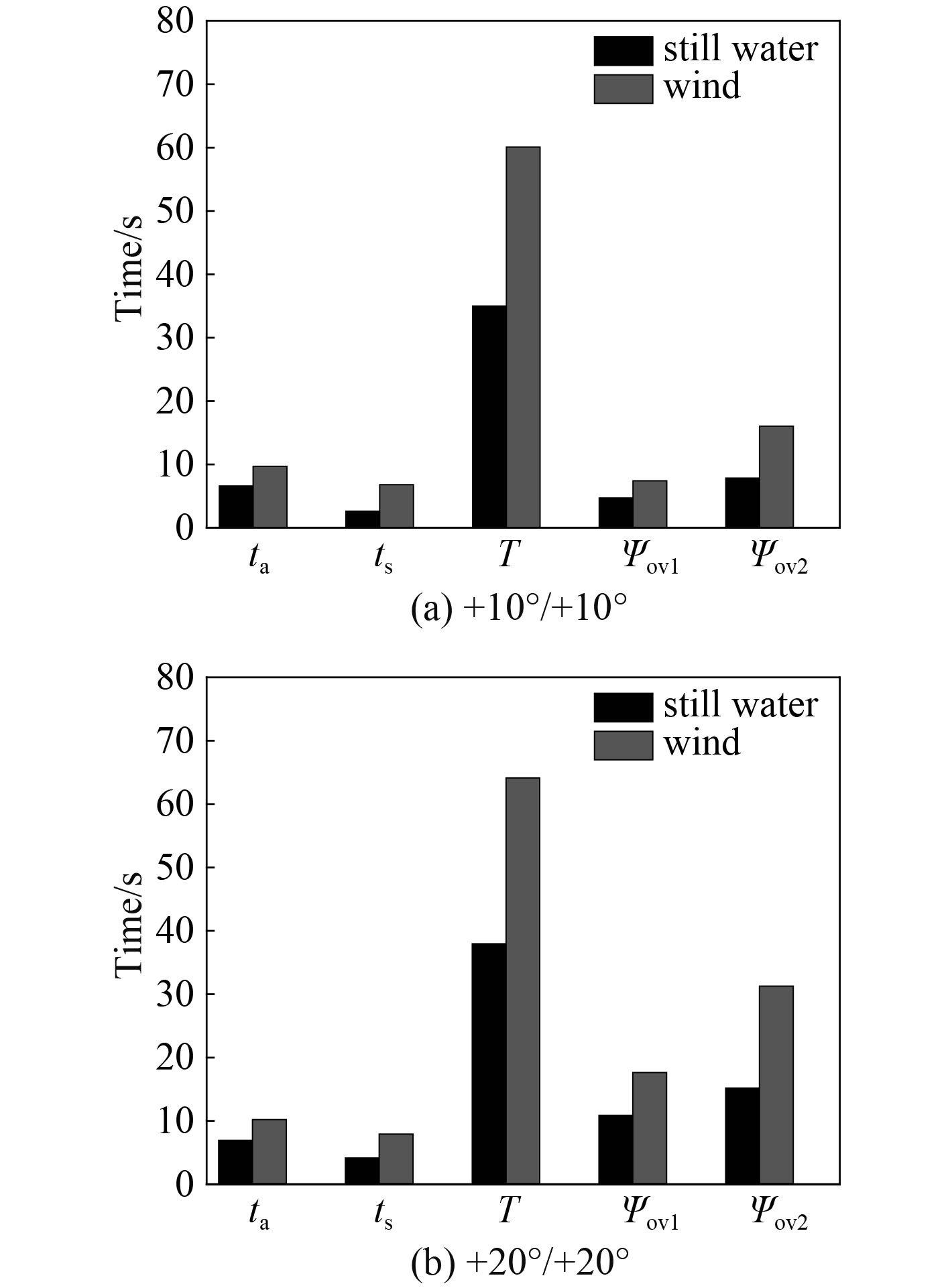图 8 不同舵角和首向角Z形特征参数的对比 Fig. 8 Comparision of zigzag characteristic parameters with different rudder angle and course angle

4 结　语

1）基于三自由度的MMG操纵性方程，对静水无风载荷的操纵性进行了数值理论预报，并与试验结果进行了误差对比分析，验证了该理论预报方法的可行性；

2）构建考虑风载荷的三自由度MMG操纵性方程，对考虑风载荷的操纵性也进行了数值理论预报，并与静水中的理论预报值进行了对比。研究发现，对于回转运动，无因次回转直径、无因次战术直径、速降、无因次横距等回转运动参数都要小于静水中理论预报值，回转角速度基本与静水理论预报值一致，无因次纵距要大于静水中的理论预报值；而对于Z形运动，初转周期、超越时间、第一超越角以及第二超越角等Z形运动参数都要大于静水中理论预报值，由此可知风载荷对该水面舰船的操纵性有一定的影响。

  朱齐丹, 于瑞亭, 夏桂华, 等. 风浪流干扰及参数不确定欠驱动船舶航迹跟踪的滑模鲁棒控制[J]. 控制理论与应用, 2007, 29(7): 35-40.  李美青, 吴秀恒. 船舶在风浪流及浅水域中多工况操纵运动模拟计算[J]. 中国造船, 1989: 38-52.  田超. 风浪流作用下船舶操纵运动的仿真计算[D]. 武汉: 武汉理工大学, 2003: 55-70.  吉春正. 风浪流环境中滑行艇操纵性研究[D]. 哈尔滨: 哈尔滨工程大学, 2007: 58-71.  TSUTOMU H, YAN L, YUTAKA H. Wind tunnel tests on a different phase three-stage savonius rotor[C]//JSME International Journal Series B Fluids and Thermal Engineering. 2005, 48(1): 9-16.  YOZO F, KICHIROK, HIROSHI T. Wind tunnel tests[J]. Wind Resistant Design of Bridges, 2012, 89-118.  BAHAJ A, MOLLAND J, CHAPLIN W. Power and thrust measurements of marine current turbines under various hydrodynamic flow conditions in a cavitation tunnel and a towing tank[J]. Renewable Energy, 2007, 32(3): 407-726. DOI:10.1016/j.renene.2006.01.012  BAHAJ A, BATTEN G. Experimental verifications of numerical predictions for the hydrodynamic performance of horizontal axis marine current turbines[J]. Renewable Energy, 2007, 32(15): 2479-2490. DOI:10.1016/j.renene.2007.10.001  FAHD F, CHRISTIAN K, ARJEN K. Predicting loads on LNG carrier with CFD[C]//Proceeding of the ASME 2010 29th International Conference on Ocean, Offshore and Arctic Engineering(OMEA 2010-2012), Shanghai, 2010.  POPINET S, SMITH M, STEVEN C. Experimental and numerical study of the turbulence characteristics of air flow around a research vessel[J]. Journal of Atmos-pheric and Oceanic Technology, 2004, 21(10): 1575-1589. DOI:10.1175/1520-0426(2004)021<1575:EANSOT>2.0.CO;2  BRIZZOLARA S, RIZZUTO E. Wind heeling moments on very large ships: Some insights through CFD results[C]// Proceeding of the 9th International Conference of Ships and Ocean Vehicles, Rio de Janeiro: Lamce Press, 2006 : 1-13.  GUILHERME V, OLAF J W, HARALD O. Semiempirical predictions and CFD computation for current coefficients of semi-submersibles[C]//Proceeding of the 28th International Conference on Ocean Offshore and Arctic Engineering, Honolulu, 2009.  JAVAHERCHI T. Review of spalar all marasturbulance modle and its modifcations[D]. Washington: ME Department, University of Washington, 2010.  XUE Y, KHARE M, CHAUDHRY K K. Wind tunnel simulation studies on dispersion at urban street canyons and insections:a review[J]. Journal of Wind Engineering and Industrial Aerodynamics, 2005, 93(9): 697-717. DOI:10.1016/j.jweia.2005.04.002  FUJIWARA T, YUKAWA K, SATO H, et al. Wind effect estimation in side by side offloading operation for FLNG and LNG carrier ships[C]//31st ASME International Conference on Ocean, Offshore and Arctic Engineering, Rio de Janeiro, BRAZIL, 2012: 71-78.  FUJIWARA T, YUKAWA K, SATO H. Wind Effect estimation and navigation-al effect in side by side offloading operation for FLNG and LNG carrier ships[C]//Offshore Technology Conference, Houston, Texa-s, USA, 2012: 23-38.  RAMAN-NAIR W, GASH R. Effect of wind and current on course control of a maneuvering vessel[C]//Oceans Conference, St Johns, CANADA, 2014: 14-19.  王化明, 邹早健. 双桨双舵船舶操纵性预报研究[J]. 武汉理工大学学报(交通科学与工程版), 2006, 30(1): 124-127. DOI:10.3963/j.issn.2095-3844.2006.01.034